# How to Create DataFrame from Dictionary in Python Pandas?

To create DataFrame from a python dictionary in Pandas, there may be several ways but here we are using mainly two ways:

1. DataFrame constructor

2. from_dict() method

The DataFrame's Constructor and from_dict() method both returns DataFrame from the provided dictionary. In this tutorial, we are discussing the conversion of python dictionary to DataFrame in Pandas with several examples.

## Pandas DataFrame Constructor

DataFrame is used to represent data in tabular format. The DataFrame constructor has the following syntax:

``DataFrame(data=None, index: Optional[Collection] = None, columns: Optional[Collection] = None, dtype: Optional[Union[str, numpy.dtype, ExtensionDtype]] = None, copy: bool = False)``

## Converting Dictionary To DataFrame in Pandas

Here, in this example, a dictionary is passed to DataFrame's constructor that is used to create a DataFrame and then we print it to the console so that we can see the desired result. See It returns data in tabular format.

``````import pandas as pd
# Take a python dictionary
data = {
'name' : ['Rohan', 'Anil', 'John'],
'age' : [30, 18, 40],
'city' : ['Tokyo', 'New Delhi', 'New york']
}
# Converting dictionary to DataFrame
dic = pd.DataFrame(data)    # DataFrame Constructor
print(dic)``````

Output: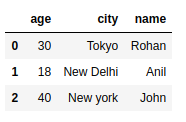## Jupyter Notebook

If you are using Jupyter notebook, then you can execute the above example like the below screenshot. If you are not familiar with Jupyter then skip this step and move and scroll down the page.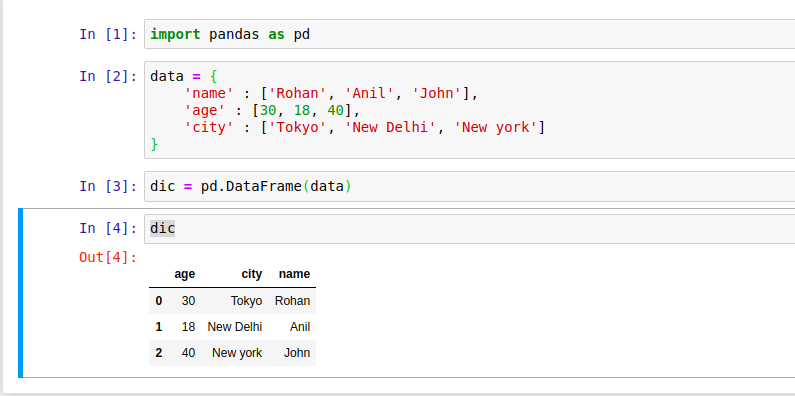By default, the keys of the dictionary become the DataFrame columns, and each row represented by a numerical value starting from 0. In the output, notice the name, age and city become column names.

Although we can set new values for the row index by passing index parameter in the DataFrame's constructor. It will set rows number 1,2 and 3 than 0, 1, and 2 as did in the above example. See the example below.

``````import pandas as pd
# Take a python dictionary
data = {
'name' : ['Rohan', 'Anil', 'John'],
'age' : [30, 18, 40],
'city' : ['Tokyo', 'New Delhi', 'New york']
}
# Converting dictionary to DataFrame
dic = pd.DataFrame(data, index=[1,2,3])    # DataFrame Constructor
print(dic)``````

Output: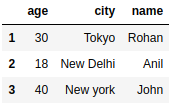## Pandas DataFrame from_dict() Method

It is a member method of DataFrame and used to create a DataFrame from a dictionary. The syntax of the method is given below.

``from_dict(data, orient='columns', dtype=None, columns=None)``

In this example, we are using the from_dict() method to get DataFrame by passing a dictionary as an argument.

``````import pandas as pd
# Take a python dictionary
data = {
'name' : ['Rohan', 'Anil', 'John'],
'age' : [30, 18, 40],
'city' : ['Tokyo', 'New Delhi', 'New york']
}
# Converting dictionary to DataFrame
dic = pd.DataFrame.from_dict(data) # DataFrame from_dict() Method
print(dic)``````

Output: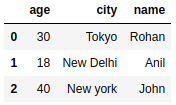## Example

By default, the keys of the dictionary become the DataFrame columns. So, If we want to change it, then pass orient="index"as the second argument to from_dict() method that will transpose the DataFrame.

``````import pandas as pd
# Take a python dictionary
data = {
'name' : ['Rohan', 'Anil', 'John'],
'age' : [30, 18, 40],
'city' : ['Tokyo', 'New Delhi', 'New york']
}
# Converting dictionary to DataFrame
dic = pd.DataFrame.from_dict(data, orient="index") # Setting index
print(dic)``````

Output: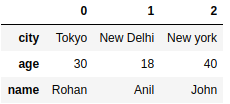After setting index orient, the columns' names are set by default from 0. We can set columns for the DataFrame by setting columns values and passing as the third argument.

## Example: Setting Columns for DataFrame

In this example, we set columns by passing columns values as an argument to the from_dict() method. Here, data represents the dictionary, orient represents the index value and columns represent column values for DataFrame.

``````import pandas as pd
# Take a python dictionary
data = {
'name' : ['Rohan', 'Anil', 'John'],
'age' : [30, 18, 40],
'city' : ['Tokyo', 'New Delhi', 'New york']
}
# Converting dictionary to DataFrame
dic = pd.DataFrame.from_dict(data, orient="index", columns=[1,2,3]) # passing column values
print(dic)``````

Output: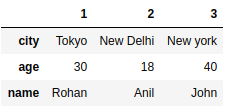## Example: Pandas DataFrame from_records() Method

This is another way to get DataFrame from the dictionary. The from_records() method belongs to DataFrame and used to get DataFrame from a list of tuples, dictionary, or DataFrame.

``````import pandas as pd
# Take a python dictionary
data = {
'name' : ['Rohan', 'Anil', 'John'],
'age' : [30, 18, 40],
'city' : ['Tokyo', 'New Delhi', 'New york']
}
# Converting dictionary to DataFrame
dic = pd.DataFrame.from_records(data) # DataFrame from_records() Method
print(dic)``````

Output: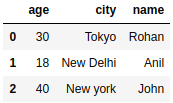### Conclusion

Well, in this topic, we learnt to create DataFrame from Python dictionary. We used DataFrame's constructor and from_dict() method to convert python dictionary to DataFrame. We explained the topic with the help of several examples.

If we missed something, you can suggest us at - [email protected]

Trending